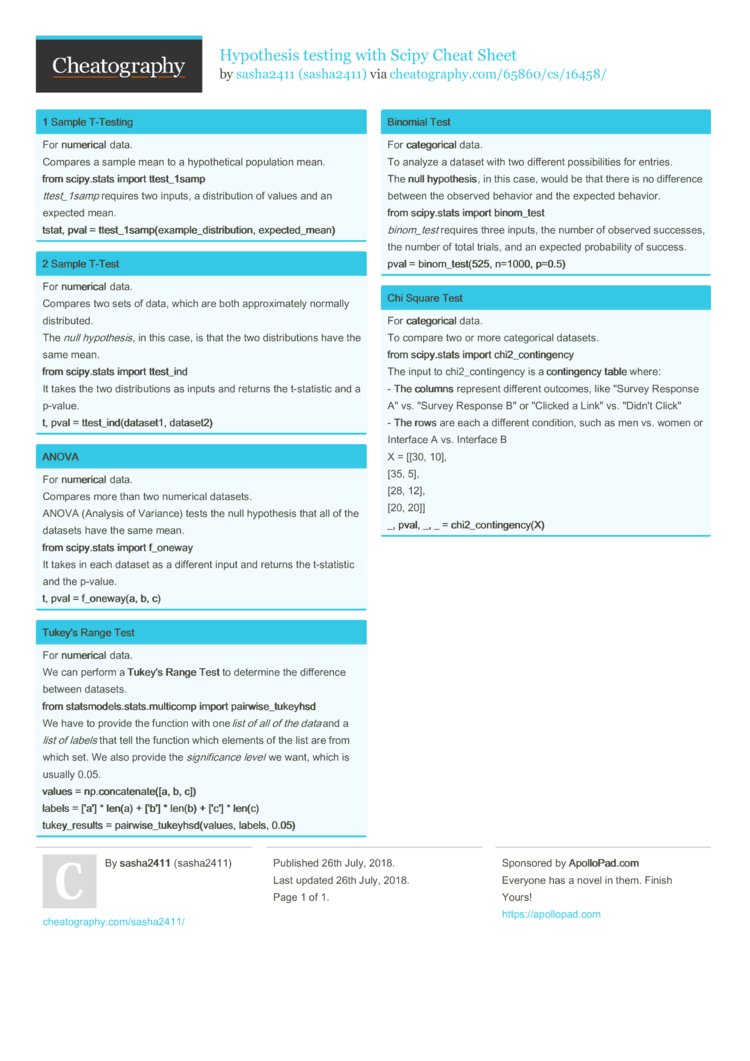# Hypothesis testing with Scipy Cheat Sheet by sasha2411

### 1 Sample T-Testing

 For nume­rical data. Compares a sample mean to a hypoth­etical population mean. from scipy.s­tats import ttest_­1samp ttest­_1samp requires two inputs, a distri­bution of values and an expected mean. tstat, pval = ttest_­1sa­mp(­exa­mpl­e_d­ist­rib­ution, expect­ed_­mean)

### 2 Sample T-Test

 For nume­rical data. Compares two sets of data, which are both approx­imately normally distri­buted. The null hypoth­esis, in this case, is that the two distri­butions have the same mean. from scipy.s­tats import ttest_­ind It takes the two distri­butions as inputs and returns the t-stat­istic and a p-value. t, pval = ttest_­ind­(da­taset1, datase­t2)

### ANOVA

 For nume­rical data. Compares more than two numerical datasets. ANOVA (Analysis of Variance) tests the null hypothesis that all of the datasets have the same mean. from scipy.s­tats import f_oneway It takes in each dataset as a different input and returns the t-stat­istic and the p-value. t, pval = f_onew­ay(a, b, c)

### Tukey's Range Test

 For nume­rical data. We can perform a Tukey's Range Test to determine the difference between datasets. from statsm­ode­ls.s­ta­ts.m­ul­ticomp import pairwi­se_­tuk­eyhsd We have to provide the function with one list of all of the data and a list of labels that tell the function which elements of the list are from which set. We also provide the signi­ficance level we want, which is usually 0.05. values = np.con­cat­ena­te([a, b, c]) labels = ['a'] * len(a) + ['b'] * len(b) + ['c'] * len(c) tuke­y_r­esults = pairwi­se_­tuk­eyh­sd(­values, labels, 0.05)

### Binomial Test

 For cate­gor­ical data. To analyze a dataset with two different possib­ilities for entries. The null hypoth­esis, in this case, would be that there is no difference between the observed behavior and the expected behavior. from scipy.s­tats import binom_­test binom­_test requires three inputs, the number of observed successes, the number of total trials, and an expected probab­ility of success. pval = binom_­tes­t(525, n=1000, p=0.5)

### Chi Square Test

 For cate­gor­ical data. To compare two or more catego­rical datasets. from scipy.s­tats import chi2_c­ont­ing­ency The input to chi2_c­ont­ingency is a cont­ingency table where: - The columns represent different outcomes, like "­Survey Response A" vs. "­Survey Response B" or "­Clicked a Link" vs. "­Didn't Click" - The rows are each a different condition, such as men vs. women or Interface A vs. Interface B X = [[30, 10], [35, 5], [28, 12], [20, 20]] _, pval, _, _ = chi2_c­ont­ing­enc­y(X)1 Page
//media.cheatography.com/storage/thumb/sasha2411_hypothesis-testing-with-scipy.750.jpg

PDF (recommended)